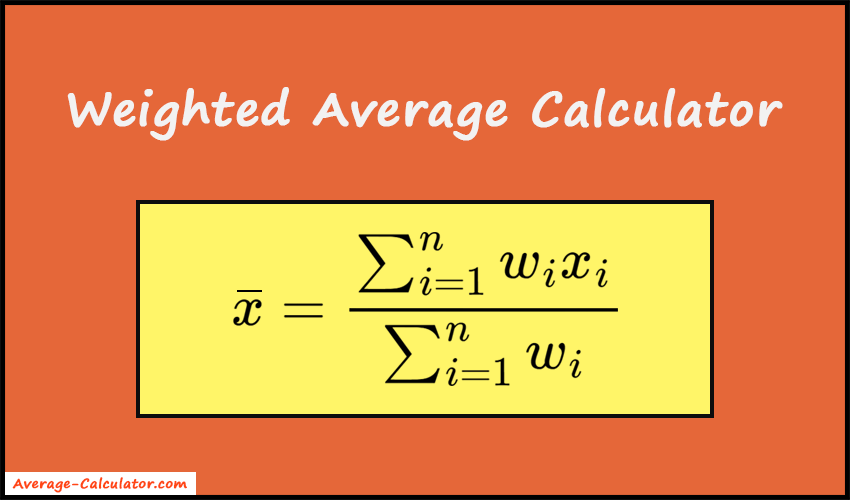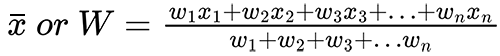# Weighted Average Calculator

Weighted Average Calculator is a free online tool that displays the weighted average or mean for the given range of values.

 Weight Data value
##### Related Tools

Welcome to our Weighted Average Calculator. It allows you to calculate the weighted average and sum of weights based on the entered data value and weights. Also, it shows the calculation steps. So, you can easily understand how the average result came.### Weighted Average Formula##### ORWhere,
x̄ or W = Weighted average
n = Number of terms to be averaged
wi = Weights applied to x values
xi = Data values to be averaged